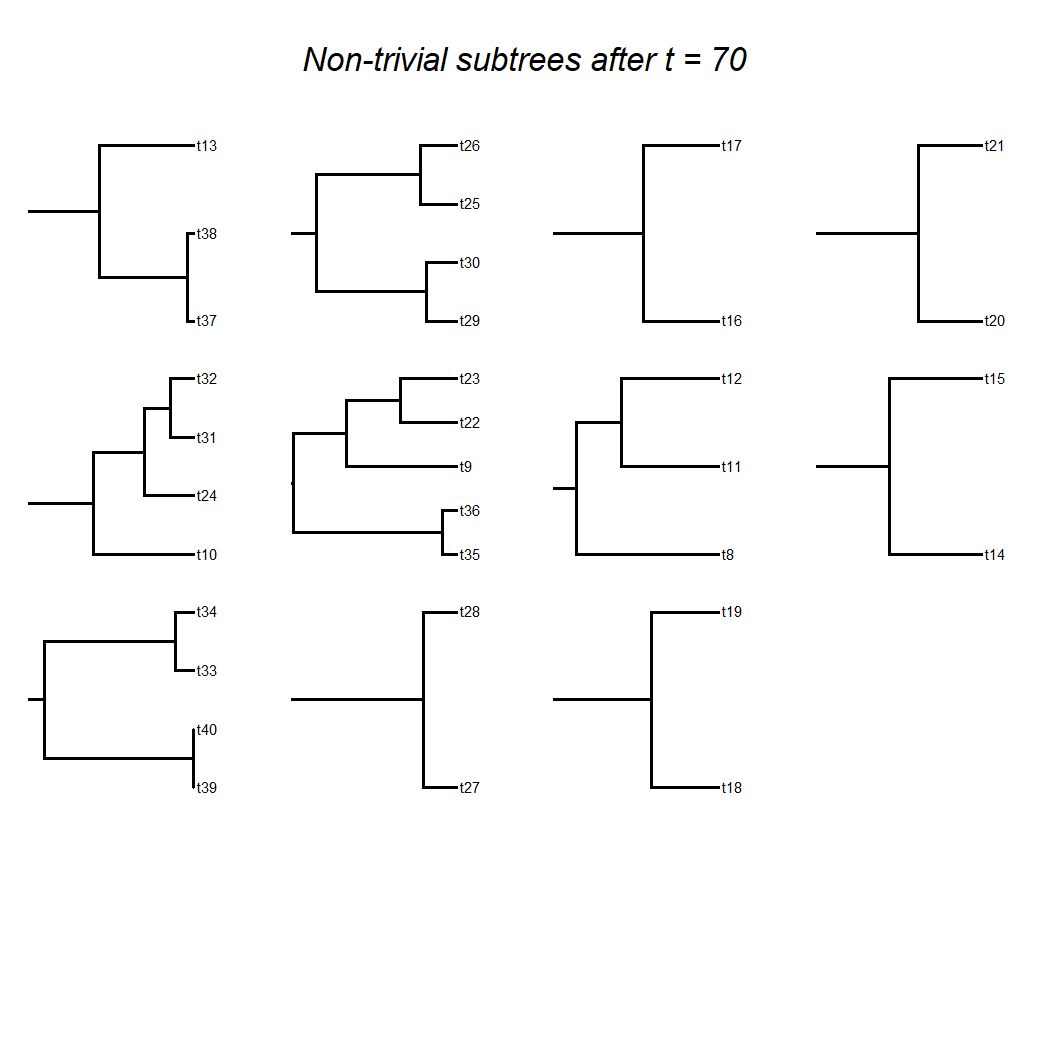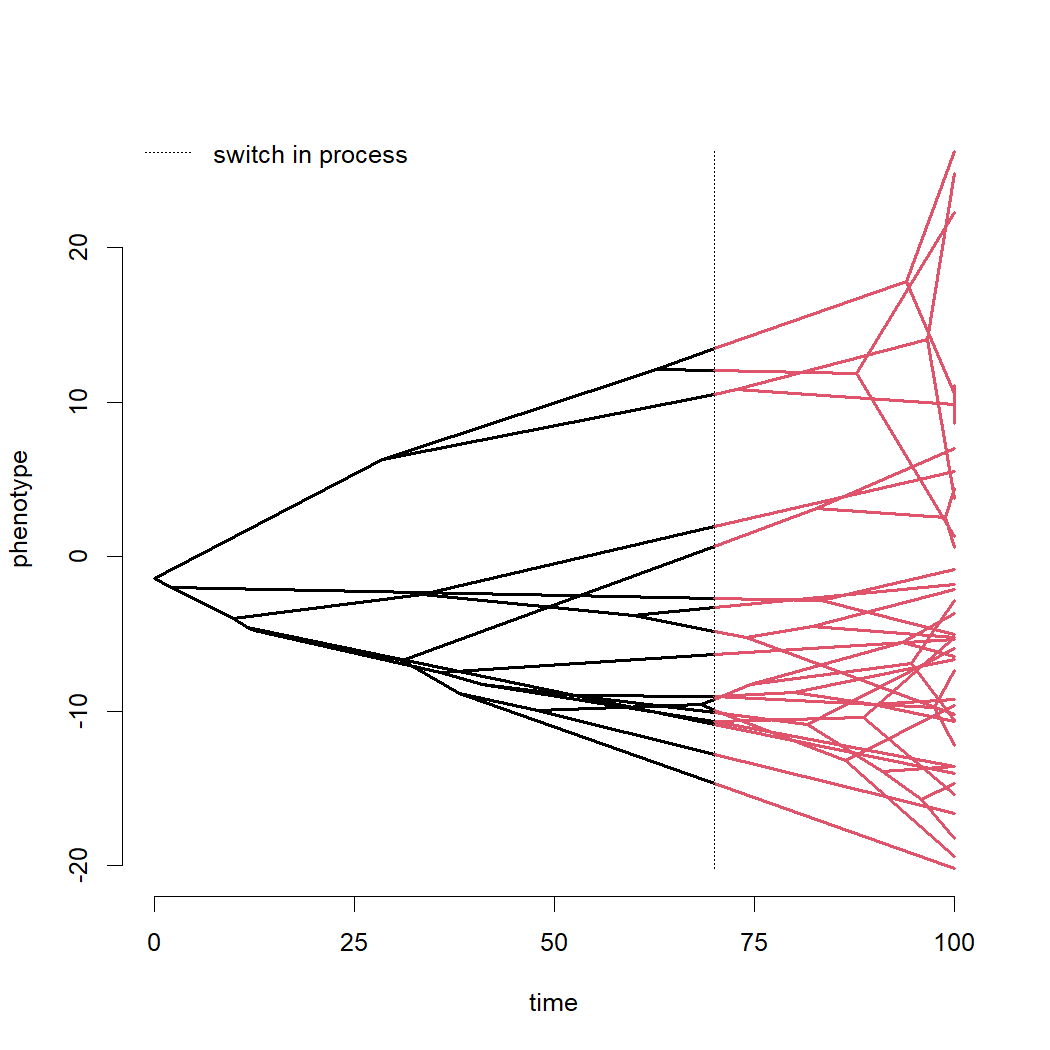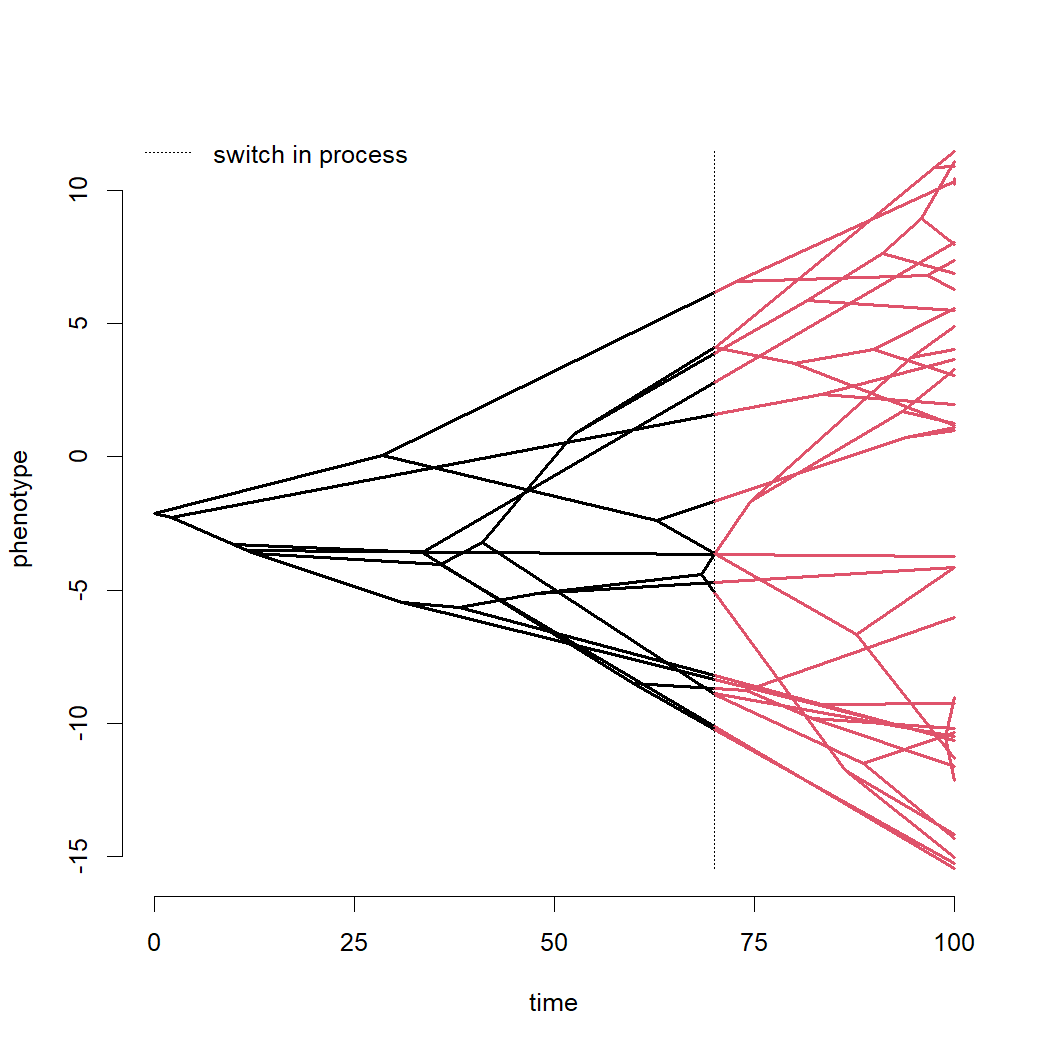## Sunday, November 7, 2021

### Simulating different phylogenetic signal before & after a specified timepoint

A few days ago, an R-SIG-Phylo user asked:

“Is there any way to simulate the evolution of a trait with a given significant signal (say K=1) from the tree root to time x and no signal (K=0) from time X to the present?”

Well, there's actually no straightforward way to simulate a predetermined value of Blomberg et al.'s (2003); however, it is possible to simulate data in which evolution in one part of the tree is by a phylogenetic signal preserving process (e.g., Brownian motion), while evolution in a different part of the tree is by a phylogenetic signal destroying process.

The way I thought of to do this was using the phytools function treeSlice.

treeSlice cuts the tree at a user-specified point (it even has an interactive mode!) and then returns either the tree up to the point of the cut (for orientation="rootwards") or all the subtrees descended from the cut (for orientation="tipwards").

My idea was to simply simulate under Brownian motion on the rootward tree, pass the simulated “tip” values from this step as root states to all the descendant subtrees, and then simulate under a $$\lambda$$ model (with $$\lambda$$ = 0, in this case) for each subtree.

Let's try it.

## load packages & simulate tree
library(phytools)
tree<-pbtree(n=40,scale=100)
## get hidden lambdaTree function (this is just to simulate random
## values for the subtrees from time t
lt<-phytools:::lambdaTree
## set t (here I'm imagining the tree has a total length of 100 and
## I want to slice it at time = 70 units above the root
t<-70
## slice the tree rootward of time = t -- this generates an object
## of class "multiPhylo"
rootward<-treeSlice(tree,orientation="rootwards",slice=t)
## now slice the tree tipward of time t -- this generates an object
## of class "multiPhylo" with a length equal to the number of tips
## in rootward
tipward<-treeSlice(tree,orientation="tipwards",trivial=TRUE,
slice=t)
## convert each subtree in tipward to a "phylo" object with an
## unbranching (singleton) root node. This is for simulation.
tipward<-lapply(tipward,rootedge.to.singleton)
## all of this is just plotting and can be ommitted
nn<-sum(sapply(tipward,Ntip)>1)
cc<-ceiling(sqrt(nn))
layout(matrix(c(rep(nn+1,cc),1:nn,rep(nn+2,cc^2-nn)),
cc+1,cc,byrow=TRUE),heights=c(0.5,rep(1,cc)))
nulo<-lapply(tipward[sapply(tipward,Ntip)>1],plotTree,mar=rep(1.1,4),
fsize=0.9)
plot(NA,xlim=c(-1,1),ylim=c(-1,1),axes=FALSE)
text(0,0,paste("Non-trivial subtrees after t =",t),cex=2,font=3)
## end plotting
## simulate the ancestral states for each subtree in tipward under
## pure Brownian motion
aa<-fastBM(rootward)
## create a vector of values
xx<-vector()
## simulate on each subtree to populate it. a is the ancestral state
## for each simulation
for(i in 1:length(tipward))
xx<-c(xx,fastBM(lt(tipward[[i]],0),a=aa[i]))We can measure phylogenetic signal:

phylosig(tree,xx,method="lambda")

##
## Phylogenetic signal lambda : 0.66126
## logL(lambda) : -142.569


Let's plot our data simulated in this way using the phytools phenogram function:

phenogram(make.era.map(tree,c(0,t)),xx,ftype="off")
legend("topleft","switch in process",lty="dotted",bty="n")
clip(0,100,min(xx),max(xx))
abline(v=t,lty="dotted")For fun, let's compare this to the opposite scenario – in which no phylogenetic signal is simulated through time = 70, and Brownian motion therafter….

t<-70
rootward<-treeSlice(tree,orientation="rootwards",slice=t)
tipward<-treeSlice(tree,orientation="tipwards",trivial=TRUE,
slice=t)
tipward<-lapply(tipward,rootedge.to.singleton)
aa<-fastBM(lt(rootward,0))
xx<-vector()
for(i in 1:length(tipward))
xx<-c(xx,fastBM(tipward[[i]],a=aa[i]))
phylosig(tree,xx,method="lambda")

##
## Phylogenetic signal lambda : 1.00018
## logL(lambda) : -123.278

phenogram(make.era.map(tree,c(0,t)),xx,ftype="off")
legend("topleft","switch in process",lty="dotted",bty="n")
clip(0,100,min(xx),max(xx))
abline(v=t,lty="dotted")